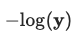• ## Binary Cross-Entropy Loss

Binary Cross-Entropy (BCE), also known as log loss, is a loss function used in binary or multi-label machine learning training.

It's nearly identical to Negative …

• ## Categorical Cross-Entropy Loss

Categorical Cross-Entropy Loss Function, also known as Softmax Loss, is a loss function used in multiclass classification model training. It applies the Softmax Activation Function …

• ## Negative Log-LikelihoodNegative log-likelihood is a loss function used in multi-class classification.

Calculated as $-log(\textbf{y})$, where $\mathbf{\text{y …}}$

• ## Mean Absolute Difference - L1 Loss

Mean Absolute Difference (MAE) is a function for assessing Regression predictions. It's also as L1 Loss because it takes the L1 Norm of the error …

• ## Root mean-squared error - L2 Loss

Root mean-squared error (RMSE) is a function for assessing Regression predictions. Sometimes called L2 Error because it takes the L2 Norm of the error vector …